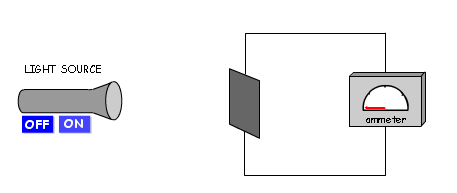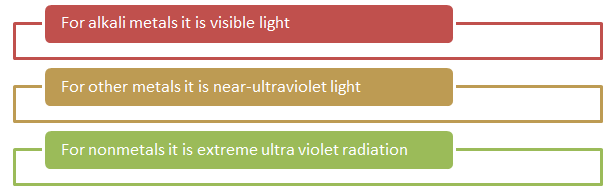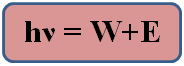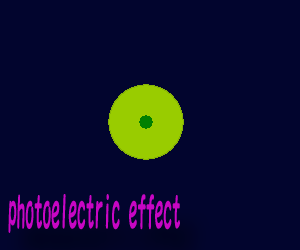×#### Thank you for registering.

One of our academic counsellors will contact you within 1 working day.

Click to Chat

1800-1023-196

+91-120-4616500

CART 0

• 0

MY CART (5)

Use Coupon: CART20 and get 20% off on all online Study Material

ITEM
DETAILS
MRP
DISCOUNT
FINAL PRICE
Total Price: Rs.

There are no items in this cart.
Continue Shopping• Complete Physics Course - Class 11
• OFFERED PRICE: Rs. 2,968
• View Details

Photoelectric Effect

Table of Content

Einstein and the Photoelectric Effect

Experimental Set up to Study Photoelectric Effect

Related ResourcesThe photoelectric effect was originally observed in 1939 and was documented by Heinrich Hertz in 1887. Initially, it was termed as the Hertz effect but later it was called the photoelectric effect.

In 1880 Hertz and Lenard observed that when a clean metallic surface is irradiated by monochromatic light of proper frequency, electrons are emitted from it. This phenomenon of ejection of the electrons from metal surface was called as Photoelectric Effect. The electrons thus ejected were called as photoelectrons. For photoemission to take place, energy of incident light photons should be greater than or equal to the work function of the metal.

Or  E ≥ W

hf ≥ W     [Where h is plank’s constant]

f ≥ W/h

Here W/h is the minimum frequency required for the emission of electrons. This is known as the threshold frequency f.

Let us discuss the photoelectric effect

On exposing a surface to a tremendous amount of energetic electromagnetic energy, it emits electrons and absorbs light. The value of threshold frequency varies according to the type of material as:The photoelectric effect crops up with photons whose energy varies from a few electron volts to over 1 MeV. Compton scattering may also happen at high proton energies comparable to electron rest energy of 511 keV.

When a light source or some electromagnetic radiation is incident upon a metallic surface, the surface can emit electrons. The electrons released in this manner are termed as photoelectrons. This is depicted in the figure given below:Einstein and the Photoelectric Effect

It was Einstein who first suggested that light consists of quanta which are generally known as photons. He proposed that the energy in each quantum of light was equal to the frequency multiplied by a constant (Planck's constant). He also suggested that a photon with frequency above a certain threshold would have adequate essential for emission of single electron thus resulting in the photoelectric effect.

Einstein’s analysis of this effect has introduced various equations which hold true for both visible as well as ultraviolet light. We discuss some of these equations:

Energy of photon = energy needed to remove an electron + kinetic energy of the emitted electronHere,

h is Planck's constant.

is the frequency of the incident photon.

W is the work function, which is the minimum energy required to remove an electron from the surface of a given metal: hν0.

E is the maximum kinetic energy of ejected electrons: 1/2 mv2

0 is the threshold frequency for the photoelectric effect.

m is the rest mass of the ejected electron.

v is the speed of the ejected electron.

Watch this Video for more referencePhotoelectric effect is the phenomenon of ejection of negatively cahrged particles (electrons) due to incidence of light on metals.

Photoelectrons are ejected with an initial velocity which varies between zero and certain maximum value.

Photo-electric current falls to zero for some negative potential of opposite erlectrode. This negative potential is called stopping potential.

Stopping potential is independent of intensity of incident light.

Stopping potential depend upon frequency of incident light.

Saturating current is independent of frequency of light.

Saturation current varies directly as the intensity of incident light.

In case the incident photon’s energy is less than the work function, none of the electron is emitted.

The rate of ejection of photoelectrons is directly proportional to the intensity of incident light for a fixed frequency of incident radiation and metal.

The time gap between the incidence and emission of a photoelectron is negligible which is less than 10–9 of a second.

For every metal there is also a bound on the minimum frequency beyond which the photoelectric effect does not occur and hence photoelectrons cannot be emitted.

Beyond the prescribed threshold frequency, the frequency of the incident radiation affects the maximum kinetic energy of the emitted photoelectron but is not affected by its intensity.

Let K1 be the maximum kinetic energy of photoelectrons emitted by light of wavelength λ1 and K2 corresponding to wavelength λ2. If λ1= 2λ2 then,

(a) 2K1 = K2             (b) K1 = 2K2

(c) K1 < K2/2                  (d) K1 > 2K2

Solution:-

As we know, K1 = hc/λ1 – W         …... (1)

and  K2 = hc/λ2 – W                      …... (2)

Substituting λ1= 2λ2 in equation (1), we get,

K1 = (hc/2λ2) – W

= ½ (hc/λ2) – W = ½ (K2+W) – W

Thus, K1 = K2/2  – W/2

Or, K1<K2/2

Therefore from the above observation we conclude that, option (C) is correct.

Experimental Set up to Study Photoelectric Effect

The given set up (as shown in fig.) is used to study the photoelectric effect experimentally.In an evacuated glass tube, two zinc plates C and D are enclosed. Plate C acts as a anode and plate D acts as a photosensitive plate. Two plates are connected to a battery B and ammeter A. If the radiation is incident on the plate D through a quartz window W, electrons are ejected out of plate and current flows in the circuit. The plate C can be maintained at desired potential (+ve or -ve) with respect to plate D. With the help of this apparatus, the dependence of the photoelectric effect can be studied on the basis of the following factors:

(b) Potential difference between C and DQuestion 1:-

Concerning the photoelectric effect, which of the following is not true?

(a) For most metals, ultraviolet light is needed for the photoelectric effect to occur.

(b) Because a faint light contains very little energy, it take as few minutes before electrons are emitted from the metal it is shining upon.

(c) A bright light causes more electrons to be emitted than a faint light.

(d) Higher frequency light emits electrons with higher kinetic energies.

Question 2:-

Max Planck

(a) proposed that light consists of photons.

(b)developed a theory to explain the absorption of light by so-called black bodies.

(c) suggested that to explain the spectrum emitted by a hot object, the energy could be viewed as given off in quanta, or units of energy.

(d) linked the energy of a photon with its amplitude using Maxwell's equations.

Question 3:-

Photons

(a) possess small but significant mass.

(b) move at speeds proportional to their frequencies.

(c) lack momentum.

(d) are localized in small regions of space.

Question 4:-

Planck's constant

(a) remains unknown to this day.

(b) is the inverse of Einstein's constant.

(c) is used to find the quantum energy associated with a certain frequency of light.

(d) is not really constant since it varies from one part of the universe to another.

Question 5:-

According to our best observations, light

(a) is exclusively a wave phenomenon.

(b) is exclusively a particle phenomenon.

(c) in any particular event, exhibits either a wave nature or a particle nature, never both at the same time.

(d) has neither wave nor particle properties.Q.1
Q.2
Q.3
Q.4
Q.5

b

c

d

c

c

Related Resources

You might like to refer Nuclear Fusion.

For getting an idea of the type of questions asked, refer the  Previous Year Question Papers.### Course Features

• 101 Video Lectures
• Revision Notes
• Previous Year Papers
• Mind Map
• Study Planner
• NCERT Solutions
• Discussion Forum
• Test paper with Video Solution